A new idea to obtain clean fusion energy

13  Confinement of positive ions and electrons with a static electromagnetic field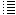A configuration with six rings (two einzel lenses)

 Image from this Wikipedia page showing a schematic drawing of an Einzel lens.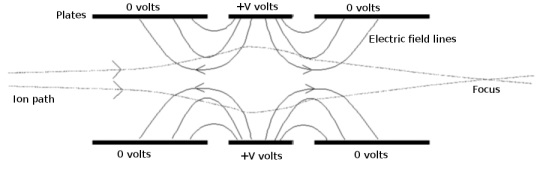Remark: According my opinion the field lines should be perpendicular near the surface of a conductor. The 0 volts rings are earthed. The positive ring will induce local charge in the earthed rings,  in order to make the electric field lines perpendicular to the surface of these earthed rings. Inside a conductor there cannot be any voltage difference = E-field.   In my simulation of the SEM fusor there are three rings. These rings can be charged with a static voltage. Actually each ring is in the program represented by 360 point charges.  Instead of a earthed ring, I will use charged rings. (it would be possible to program an earthed conductive ring, but at the moment I do not have this).   Fig. 1. Simulation of a Einzel lens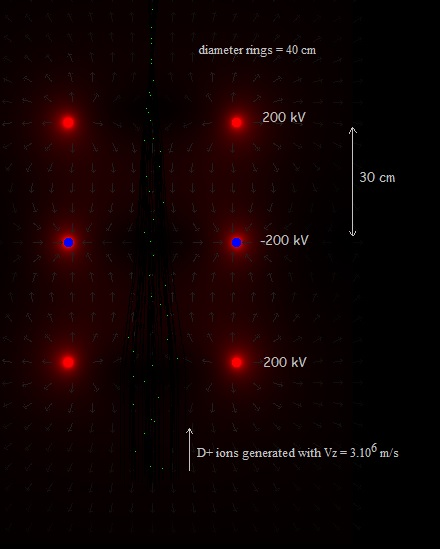D+ ions are generated with a upwards vertical speed of 3E6 m/s.  The x and y positions are random within  bounderies. In the program:  (hydrogen=deuterium ion) hydrogen[i].x:=0.5+ ( - 0.5 + random) /7;   {0,43<=x<=0,57} hydrogen[i].y:=0.5+ ( - 0.5 + random) /7;  {0,43<=y<=0,57} hydrogen[i].z:=-0.10 + ( - 0.5 + random) /100;  {not so important} hydrogen[i].m:=mp  {=2*1.6726E-27} hydrogen[i].vx:=0 ; hydrogen[i].vy:=0; hydrogen[i].vz:=0 +( - 0.5 + random)*ve/1000 + vid; {vid is introduced in the program's menu, here 3E6m/s} hydrogen[i].q:=qe; Coordinate system.jpg  (see also Droomcomputersimulation.html )   Fig. 2.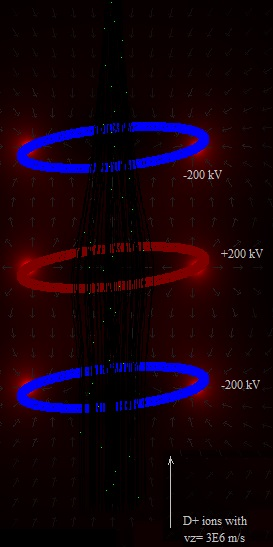The same, but wih reversed voltages. Expressing the speed of the D+ ions in a voltage difference: q.ΔV = ½ . m.v2 q = 1,6E-19 C   (charge of D+ ion) m = 2*1.6726E-27 kg (massa D+ ion) v = 3E6 m/s -> ΔV = 1,045E-8.  v2 = 94 kV  , quite high .. v=1E5 m/s ->  ΔV =   105 V v=5E5 m/s ->  ΔV =   2,6 kV v=3E5 m/s ->  ΔV =   941 V Fig. 3.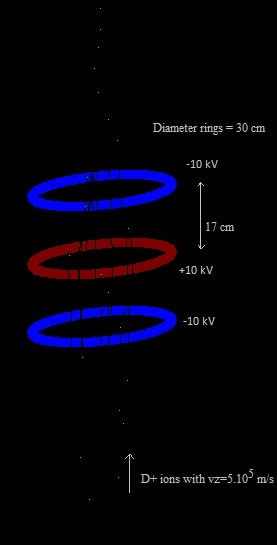Fig. 4.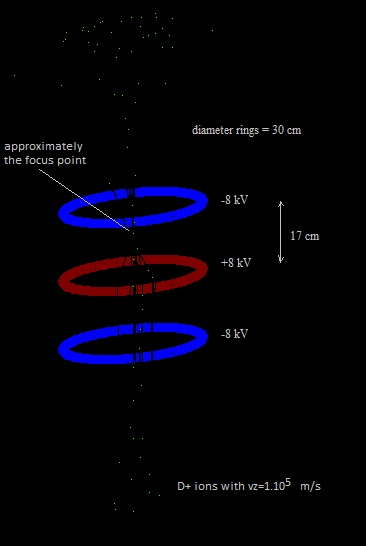Fig. 5.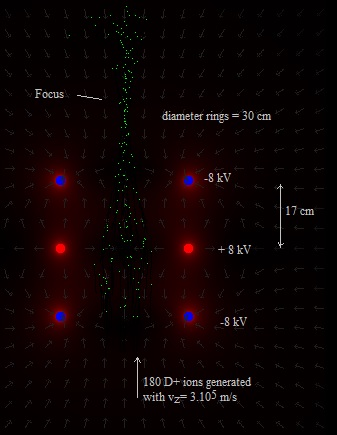Changed a little bit the sourch code so that the electric field is visible with this lower values. The direction of the arrows indicate the direction of the electric field, and the color the strength (the more red, the stronger the field). If the voltage is too high (more than about ±10 kV) then the ions will return and go downwards again. Fig. 6.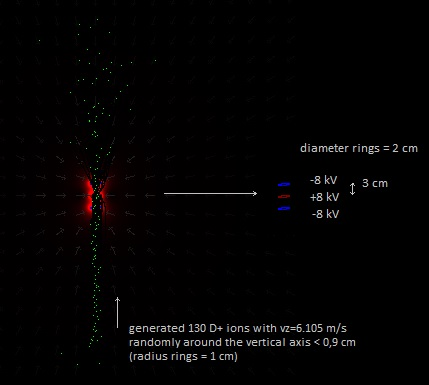vz=6E5 m/s The D+ are generated with only a upwards vertical speed, <0,9 cm from the central vertical axis. They move through the three rings of the Einzel lens. You can see there is a focus (although not very clear), after which the D+ ions diverge (spread). The visiual image could be improved.. Fig. 7. Simulation experiment.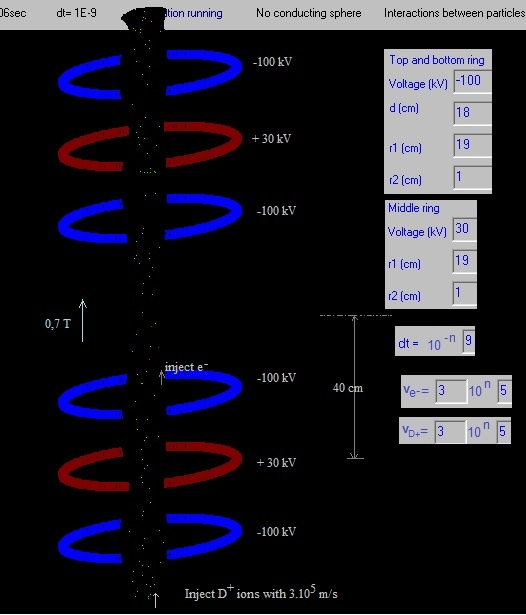D+ ions are generated with a upwards vertical speed of 3E5 m/s, 15 cm under the lowest blue ring. The x and y positions are random within  bounderies. hydrogen[i].x:=0.5+ ( - 0.5 + random) /16;  {hydrogen=deuterium} {50 cm +/- 3,1 cm} hydrogen[i].y:=0.5+ ( - 0.5 + random) /16; hydrogen[i].z:=0.5-dr-hch-0.15 + ( - 0.5 + random) /100; De electrons are injected 8 cm above the top blue ring in the bottom half. electron[i].x:=0.50+ ( - 0.5 + random) /100; {50 cm +/- 5 cm } electron[i].y:=0.50+ ( - 0.5 + random) /100; electron[i].z:=0.50+dr-hch+0.08 + ( - 0.5 + random) /100; electron[i].vx:=0 + (-0.5 + random)*ve/1000; electron[i].vy:=0 + (-0.5 + random)*ve/1000; electron[i].vz:=0 + (-0.5 + random)*ve/1000+vie; { ve:=vid*2;}  {vid = 3E5 m/s} No focus, no einzel lens effect. It seems to be that the strong magnetic field is causing this.The electrons stay confined between the two blue rings in the center, forming a negative cloud. An advantage of this configuration could be that, if fusion occurs in the center, then this a bit further away from the rings than in the configuration with only three rings (less danger that the rings get damaged). Fig. 8. Simulation experiment with a magnetic bottle field.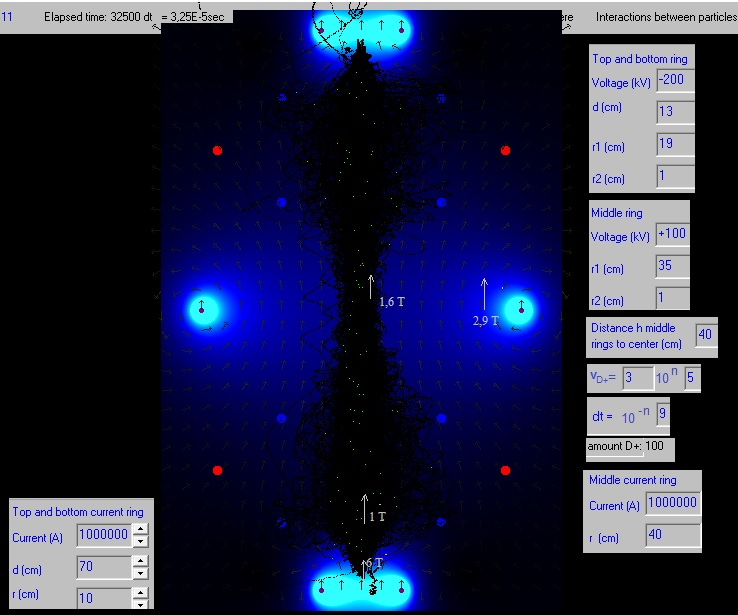Some D+ ions escaped.  The D+ ions are somewhat squezed in the center. But this is also seen in: Droom12/Droom12.htm. Probably this is an effect of the shape of the magnetic field, and not of the Einzel lens configuration. Fig. 9. Simulation experiment.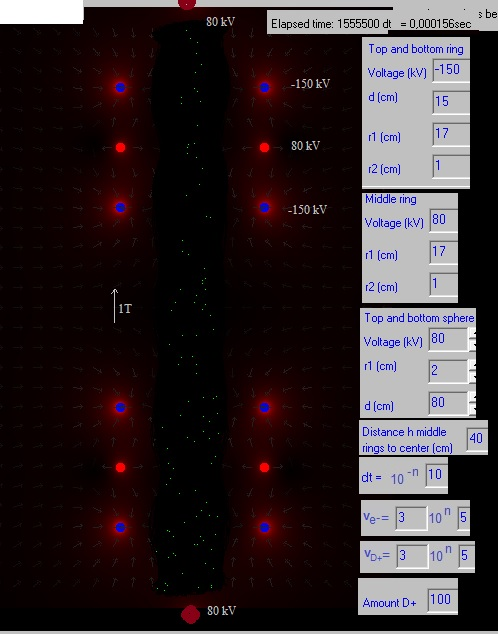Another simulation experiment. Shown is a cross section and the electric field. A little "lens effect" is seen. Fig. 9. The same simulation experiment after a night running.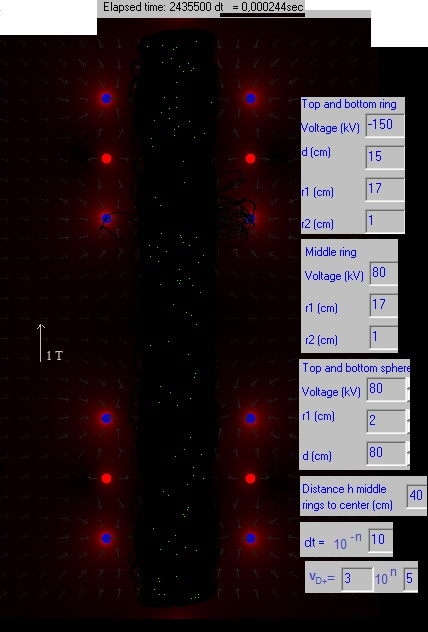2020     by  Rinze Joustra        www.valgetal.com